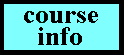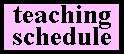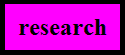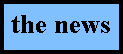Paul C. Pasles,  Villanova University Department of Mathematics and Statistics office: Saint Augustine Center 324 phone: 610-519-7345 email: paul.pasles@villanova.edu# THE BOOK

Read about Benjamin Franklin's Numbers! Check your local bookstore or order from Princeton University Press or Amazon. You can read the reviews here and the first chapter here.

PUBLICATIONS

Nonanalytic automorphic integrals on the Hecke groups. Acta Arithmetica 90:2, 1999.

Convergence of Poincaré series with two complex coweights. Contemporary Mathematics 251, 2000.

A Hecke correspondence theorem for nonanalytic automorphic integralsJ. of Number Theory 83:2, 2000.

The lost squares of Dr. Franklin. American Mathematical Monthly 108:6, 2001.     rare squares

Row coincidences in nonsingular binary matrices. European J. of Combinatorics 22:2, 2001. (With O. Marrero.)

A generalization of the Lipschitz summation formula & some applications.   Proc. Amer. Math. Society 129:11, 2001.  (With W. Pribitkin.)

Binary matrices. Elemente der Mathematik 57:4, 2002. (With O. Marrero.)

Digging for squares. Math Horizons 9:4, 2002.

Multiplier systems. Acta Arithmetica 108:3, 2003.

Coin ToGa: A coin-tossing game. College Mathematics Journal 34:3, 2003. (With O. Marrero.)

Franklin's other 8-square. Journal of Recreational Mathematics 31:3, 2003.

Some magic squares of distinction. Math Horizons 11, 2004.

A strange new prime pattern. (With O. Marrero.) Journal of Recreational Mathematics 33:4, 2005.

A bent for magic. Mathematics Magazine 31:3, 2006.

Benjamin Franklin's Numbers (book). Princeton University Press, 2008.

Markov and Coin ToGa. (With O. Marrero.)
Mathematics and Computer Education 42, 2008.

Fibonacci matrices and modular forms. Fibonacci Quarterly 48:4, 2010.

Pictorial moments of Coin ToGa. (With O. Marrero.) Mathematics and Computer Education 45, 2011.

A combinatorial problem on a directed graph. (With O. Marrero.) Rev. Mat. Teor. Apl. 45, 2016.

Convergence of Poincaré series on Hecke groups of large width. Rocky Mountain Journal of Mathematics, to appear.

The multivariate probabilistic Josephus problem. (With O. Marrero.) Submitted.

MAT 1230-1235/ Introductory Statistics I-II

MAT 1320-1325/ Calculus for Liberal Arts I-II

MAT 1330-1335/ Calculus and Quantitative Analysis I-II

MAT 1500-1505-2500/ Calculus I-II-III

MAT 2600/ Foundations of Mathematics

MAT 2705/ Differential Equations with Linear Algebra

MAT 3305/ Topics in Analysis

MAT 3400/ Linear Algebra

MAT 3500/ Modern Algebra

MAT 3930/ History of Mathematics

MAT 4110/ Combinatorics

MAT 5110/ Topics in Geometry

MAT 5200/ Theory of Numbers

MAT 5400/ Complex Analysis

MAT 7290/ Geometry

MAT 7300/ History of Mathematics

MAT 7610/ Complex Analysis

MAT 7660/ Linear Algebra

MAT 7670/ Mathematical Logic

MAT 7770/ Number Theory

MAT 8650/ Abstract Algebra

MAT 8790/ Selected Topics

more courses